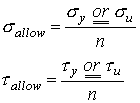Linear-Elastic Response and Factor of Safety

Linear-elastic response:

Hooke’s law:

In the linear elastic portion of the response of material one can model the response by Hooke’s law:

 Hooke’s law for extension: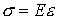Hooke’s law for shear:Where E is the elastic modulus (also known as Young’s modulus), and G is the shear modulus. The elastic and shear moduli are material constants characterizing

the stiffness of the material.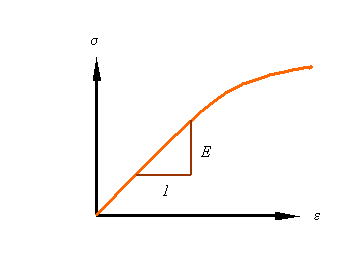Poisson’s Ratio:

Another material parameter is Poisson’s ratio that characterizes the contraction in the lateral directions when a material is extended. The symbol n (nu) is used for the poison ration, which is negative the ratio of the lateral strain to axial strain.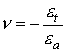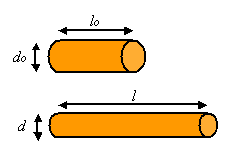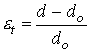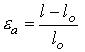Volumetric strain during extension:

During the extension of a bar the volumetric strain can be calculated as the ratio of the change in volume to the original volume. Denoting the volumetric strain as e, the initial volume as Vo and the final volume as V, one can calculate the volumetric strain from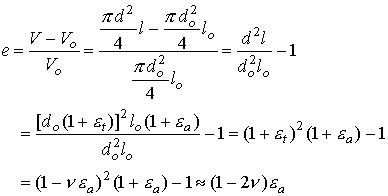The last step is contingent on the axial strain being small relative to unity.

The relation between the elastic moduli:

For an isotropic elastic material (i.e., an elastic material for which the properties are the same along all directions) there are only two independent material constants. The relation between these three moduli are given by the equation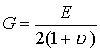Factor of safety:

The factor of safety denoted by n is the ratio of the load the structure can carry divided by the load it is required to take.Therefore, the factor of safety is a number greater than unity (n>1). The allowable stress for a given material is the maximum stress the material can take (normally the ultimate or yield stress) divided by the factor of safety.